### DatasheetsN° of component

## Shields with Diodes and Transistors (MIC030E)

Have you ever had latency problems with your design using a microcontroller? Do you need a simple logic function to connect sensors or output devices to a microcontroller and do not want to spend a lot on a shield? If these are problems you have had, are having or will one day have, then you should know simple solutions which make use of diodes and transistors.

Many people who do designs with microcontrollers, study or teach, sometimes forget that certain problems which occur with shields can have very simple solutions. Even the latency that requires the use of shields may have solutions which do not need to be bought ready.

We only remind you that latency problems occur when a microcontroller needs to read what happens with two sensors and the indication of simultaneity is fundamental.

The microcontroller processes the input data sequentially and if two sensors are only for an instant at the same state and the microcontroller reads, it does not "catch" those simultaneous states if they are too short and the result is the wrong processing.

Logic gates using diodes or transistors are simple solutions which allow you to obtain logical concurrency information so that a microcontroller needs to read only one input.

The same happens if we need to trigger a load from two simultaneous data from the outputs of a microcontroller.

In this article, we will give some interesting circuits of simple logic functions that can be elaborated with diodes and transistors.

## Practical Circuits

### a) AND gate with diodes

Our first circuit is from an AND gate using diodes. In this example, we have a three-input circuit and the diodes used can be the commonly used as a 1N4148. For 5 V (TTL) applications the resistor can be 330 ohm or 470 ohm. The circuit is shown in Figure 1.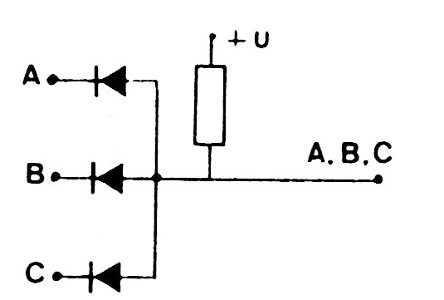Figure 1- AND gate with diodes

### b) OR gate with diodes

In Figure 2, we have the method of implementing an OR gate with diodes. The diodes can be the commonly used as the 1N4148 and the resistor will have 330 ohm or 470 ohm for TTL logic of 5 V. The number of inputs can be changed.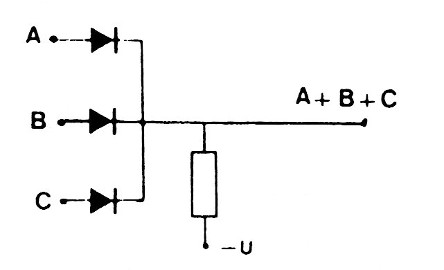Figure 2 - OR gate with diodes

### c) AND and OR function with diodes

This interesting circuit of combinational logic has the functions AND and OR. The diodes are the commonly used and the resistors can have values ??from 470 ohm to 2k2. The circuit is in Figure 3.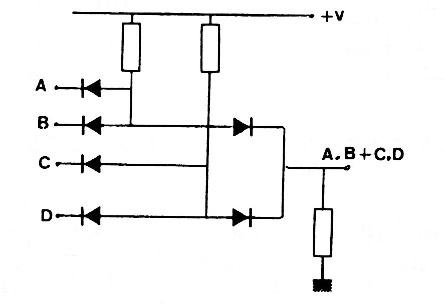Figure 3 - AND-OR function with diodes

### d) OR and AND function with diodes

The circuit of Figure 4 combines the OR and AND functions with diodes. The diodes can be the commonly used as the 1N4148 and resistors from 470 ohm to 2k2.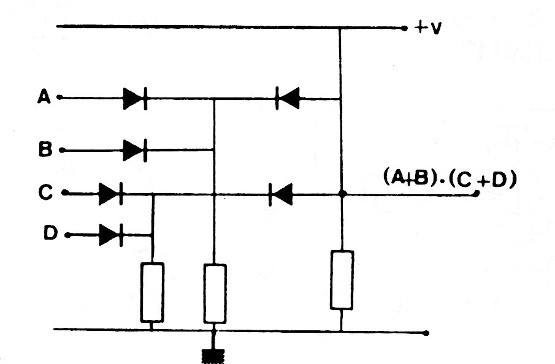Figure 4 - OR-AND function with diodes

### e) NAND gate with 1 Transistor and Diode

The circuit of Figure 5 implements a NAND logic function with diodes and transistors. In this case, we have 3 entries, because their number can be changed. The diodes are the commonly used and the transistor can be a BC548. The base resistor has 22k and the collector 2k2 for 5 V logic.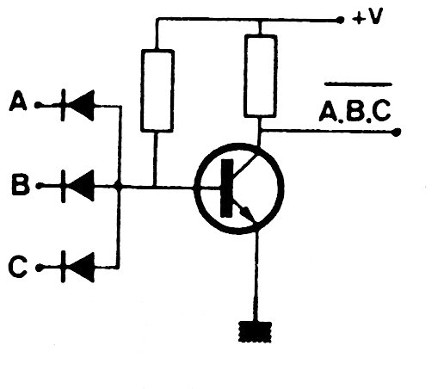Figure 5 - NAND gate with diodes

### f) NAND gate with two transistors

This gate is simpler than the previous having as collector resistor one of 2k2 for logic of 5 V. However, 1k resistors must be connected in series to the input to make the current limitation. The transistors can be the BC548.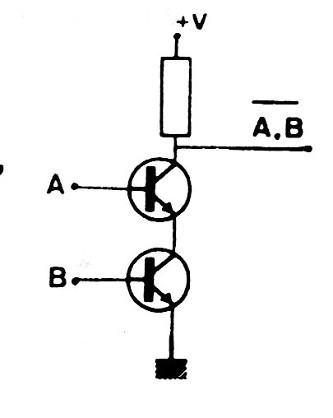Figure 6 - NAND gate with two transistors

### g) NOR gate with diodes and a transistor

The NOR gate shown in Figure 7 has three entries, but this number can be changed. The transistor can be the BC548 and the diodes are the commonly used. The collector resistor has 2k2p for 5V logic and the base resistor 47k.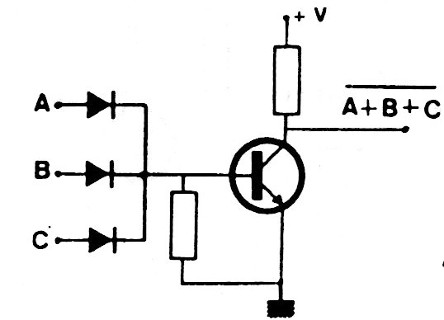Figure 7 - NOR Gate

### h) NOR function with two transistors

The NOR function with two inputs can be extended. The transistors may be the BC548, but 1k resistors in series with the current limiting inputs must be connected. The collector resistor may have 2k2.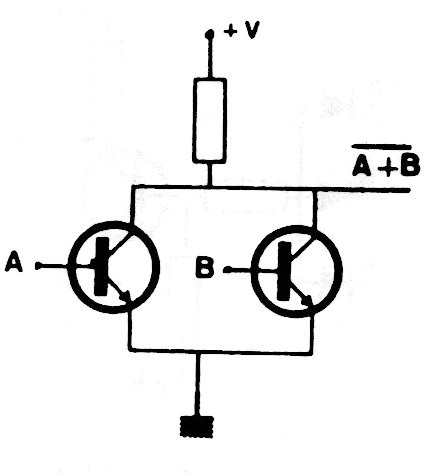Figure 8 - NOR Function

### i) Exclusive OR with diodes and transistors

We complete our sequence of simple functions with diodes and transistors with the circuit of Figure 9 of an OR-Exclusive gate using diodes and transistors. The transistors can be the BC548 and for 5V logic change the resistor from 47k to 22k, 22k to 10k and 12k to 4k4. The diodes can be 1N4148 or equivalent.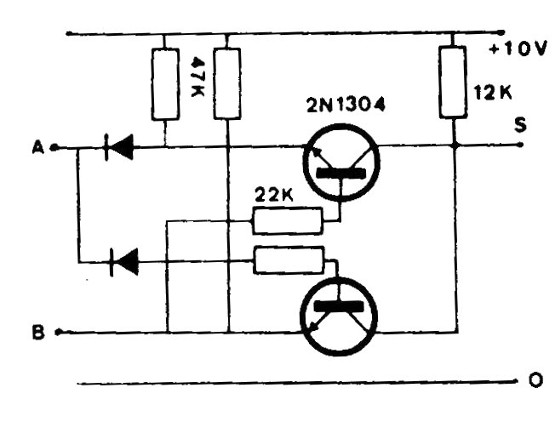Figure 9 - Or-Exclusive with diodes and transistors

## Conclusion

If you are developing your design and have a protoboard, you can perfectly test these shields before opting for an integrated solution, if you do not have it, to check if the possible cause of a problem is latency or otherwise.

We remind you that, just as latency affects many designs, coupling problems must also be considered.